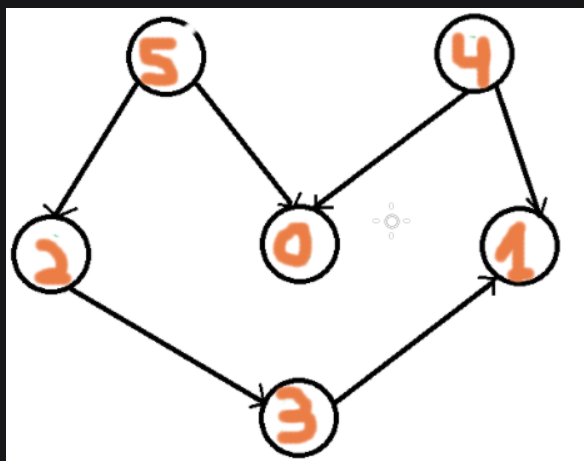# Kahn's Algorithm

Introduction

Topological sorting is Done for Directed Acyclic Graph(DAG).In which Linearing ordering of vertices is achieved where for every edge that is directed (name-UV).

Note, Here you are, the starting vertex, and v is the ending vertex.

Topological sorting handling Khan’s algorithm.

What is Kahn's Algorithm?

It is a sorting Algorithm used for sorting Topological Directed Acyclic Graph(DAG). This algorithm is applicable only in the case of a Directed Acyclic Graph(DAG).

The first vertex in the Topological Sorting of graphs is decided because the node has the In-Degree zero(i.e. the node has zero incoming nodes). One important thing that must be kept in mind is that topological sorting can have more than one sorting. For example, the below graph can have two sorting i.e

• 5 ,4 ,2 ,3 ,1 ,0,,
• 4 ,5 ,2 ,0 ,3 ,1Explanation for the output:

Topical Sorting for the Directed acyclic graph is done so that every edge begins with u (vertex ) and ends with v(vertex).In the above, Image 5 has no Incoming edge. The same scenario is with vertex 4, Whereas 0, 1 has an incoming edge only no outgoing edge.  in  0” have the incoming edges from 5,4, so one comes at last, so the order is

5,4,2,3,1,0;

Note:

A Directed Acyclic Graph has a minimum of one vertex Where Indegene Zero and OutDegree is Zero.

Algorithm:

• Step1:-Compute the Indegree of all the vertices of the Graph and Initialize the variable count with zero(It maintains the Visited node count).
• Step 2:- All the nodes with the in-degree must have zero pick them and put them in a Queue.
• Step 3:-Pick the node from the Queue mark it visited then, increment the count value and Decrement the Indegree value for all the nodes that are edges from the given node.
• Step 4:-Keep Doing step 3 until the Queue is Empty.
• Step 5:-Now compare the count value with the no of nodes of the graph,in-degree, and if they are not equal, then the Topological sort is not possible for the Graph.

How to Get In-degree for each vertex of Graph?

We deal with two major methods to find the in-degree of the vertex of the graph they are:

1. Take an in-degree array which will keep track of  indegree-of the vertex,

Traverse the edge array of the graph and increase the destination node value with the one.

``````//initialization for each node
for each node in Nodes
indegree[node] = 0;
//increment if the indegree for the node if it is the destination node
for each edge(source, destination) in Edges
indegree[destination]++;``````

Time Complexity: Since we are travelling the node first and then we are travelling the edges,, o the time complexity is o(V+E);

2. Traverse the every node of the graph and than increment it as ,How many node are connected to it and the connection is inward;

``````//traversing the every node of graph
for each nodes in Node
//finding no of incoming node to it
If (list[nodes].size()!=0) then
for each destination in lists
indegree[destination]++;``````

Time Complexity: Here we have, two loops in the two the outer loops would run (no of vertices time i.e. V), the inner loop run (no of edges time i.e. E) so the time complexity is o(V+E);

Code for the Algorithm

``````
import java.util.*;
// Class to represent a graph
class Graph {
//  vertices of the graph
int V;
// parameterised constructor
public Graph(int V)
{
this.V = V;
for (int i = 0; i < V; i++)
}
//function to create edge of graph
public void addEdge(int u, int v)
{
}
//topological sort printing
public void topologicalSort()
{
//array creation for the indegree array
int in_degree[] = new int[V];
//Filling the indegree in o(v+e) complexity
for (int i = 0; i < V; i++) {
for (int node : temp) {
in_degree[node]++;
}
}

//creating a queue and adding all the vertices with the degree 0
for (int i = 0; i < V; i++) {
if (in_degree[i] == 0)
}
int cnt = 0;
Vector<Integer> topOrder = new Vector<Integer>();
while (!q.isEmpty()) {
int u = q.poll();
for (int node : adj[u]) {
if (--in_degree[node] == 0)
}
cnt++;
}

// Check if there was a cycle
if (cnt != V) {
System.out.println("cycle is present in the graph");
return;
}
// output printing
for (int i : topOrder) {
System.out.print(i + " ");
}
}
}
class Coding_Ninjas {
public static void main(String args[])
{
Graph codingninjas = new Graph(6);
//above the the graph creation
System.out.println(" Topological Sort function will run now");
codingninjas.topologicalSort();
}
}``````

Output:What is the Complexity for the Kahn’s Algorithm?

Time Complexity:

Here we are travelling with the vertices and edges, and the outer loop is crossing, travelling the no of vertices time, so, i.e. V and the inner circle is traversing the no of Edges time, so Time Complexity is :

O(V+E);

Space Complexity:

Here we need an additional Queue for Storing all the vertices with an out-degree zero, so the Space complexity is:

O(V).

1  -What is Topological sorting?

-> Sorting Done to achieve linear ordering of Vertices

2  -What is Khan’s Algorithm?

->It is a method to achieve topological sorting for (DAG).

3  -What is DAG?

-> It is a Directed acyclic Graph in which edges have direction

4  -What is the Indegree of the graph?

-> It refers to the number of edges pointing toward itself.

5 -What is the Time and space Complexity?

->O(V+E)

## Key Takeaways:

The blog explained Topological sorting and how topological sorting is achieved using Kahn’s algorithm.